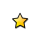On-line GuidesAll GuideseBook StoreiOS / AndroidLinux for BeginnersOffice ProductivityLinux InstallationLinux SecurityLinux UtilitiesLinux VirtualizationLinux KernelSystem/Network AdminProgrammingScripting LanguagesDevelopment ToolsWeb DevelopmentGUI Toolkits/DesktopDatabasesMail SystemsopenSolarisEclipse DocumentationTechotopia.comVirtuatopia.comAnswertopia.comHow To GuidesVirtualizationGeneral System AdminLinux SecurityLinux FilesystemsWeb ServersGraphics & DesktopPC HardwareWindowsProblem SolutionsPrivacy PolicyOpenOffice Calc 3.x Guide
Previous Page Home Next Page

You can access the OOo internal objects directly to manipulate a Calc document. For example, the macro in Listing 7 adds the values in cell A2 from every sheet in the current document. ThisComponent is set by StarBasic when the macro starts to reference the current document. A Calc document contains sheets: ThisComponent.getSheets(). Use getCellByPosition(col, row) to return a cell at a specific row and column.

Listing 7. Add cell A2 in every sheet.

``` ```

``` Function SumCellsAllSheets() Dim TheSum As Double Dim i As integer Dim oSheets Dim oSheet Dim oCell oSheets = ThisComponent.getSheets() For i = 0 To oSheets.getCount() - 1 oSheet = oSheets.getByIndex(i) oCell = oSheet.getCellByPosition(0, 1) ' GetCell A2 TheSum = TheSum + oCell.getValue() Next SumCellsAllSheets = TheSum End Function ```A cell object supports the methods getValue(), getString(), and getFormula() to get the numerical value, the string value, or the formula used in a cell. Use the corresponding set functions to set appropriate values.

Use oSheet.getCellRangeByName("A2") to return a range of cells by name. If a single cell is referenced, then a cell object is returned. If a cell range is given, then an entire range of cells is returned (see Listing 8). Notice that a cell range returns data as an array of arrays, which is more cumbersome than treating it as an array with two dimensions as is done in Listing 5.

Listing 8. Add cell A2:C5 in every sheet

``` ```

``` Function SumCellsAllSheets() Dim TheSum As Double Dim iRow As Integer, iCol As Integer, i As Integer Dim oSheets, oSheet, oCells Dim oRow(), oRows() oSheets = ThisComponent.getSheets() For i = 0 To oSheets.getCount() - 1 oSheet = oSheets.getByIndex(i) oCells = oSheet.getCellRangeByName("A2:C5") REM getDataArray() returns the data as variant so strings REM are also returned. REM getData() returns data data as type Double, so only REM numbers are returned. oRows() = oCells.getData() For iRow = LBound(oRows()) To UBound(oRows()) oRow() = oRows(iRow) For iCol = LBound(oRow()) To UBound(oRow()) TheSum = TheSum + oRow(iCol) Next Next Next SumCellsAllSheets = TheSum End Function ```When a macro is called as a Calc function, the macro cannot modify any value in the sheet from which the macro was called.

OpenOffice Calc 3.x Guide
Previous Page Home Next PagePublished under the terms of the Creative Commons License Design by Interspire